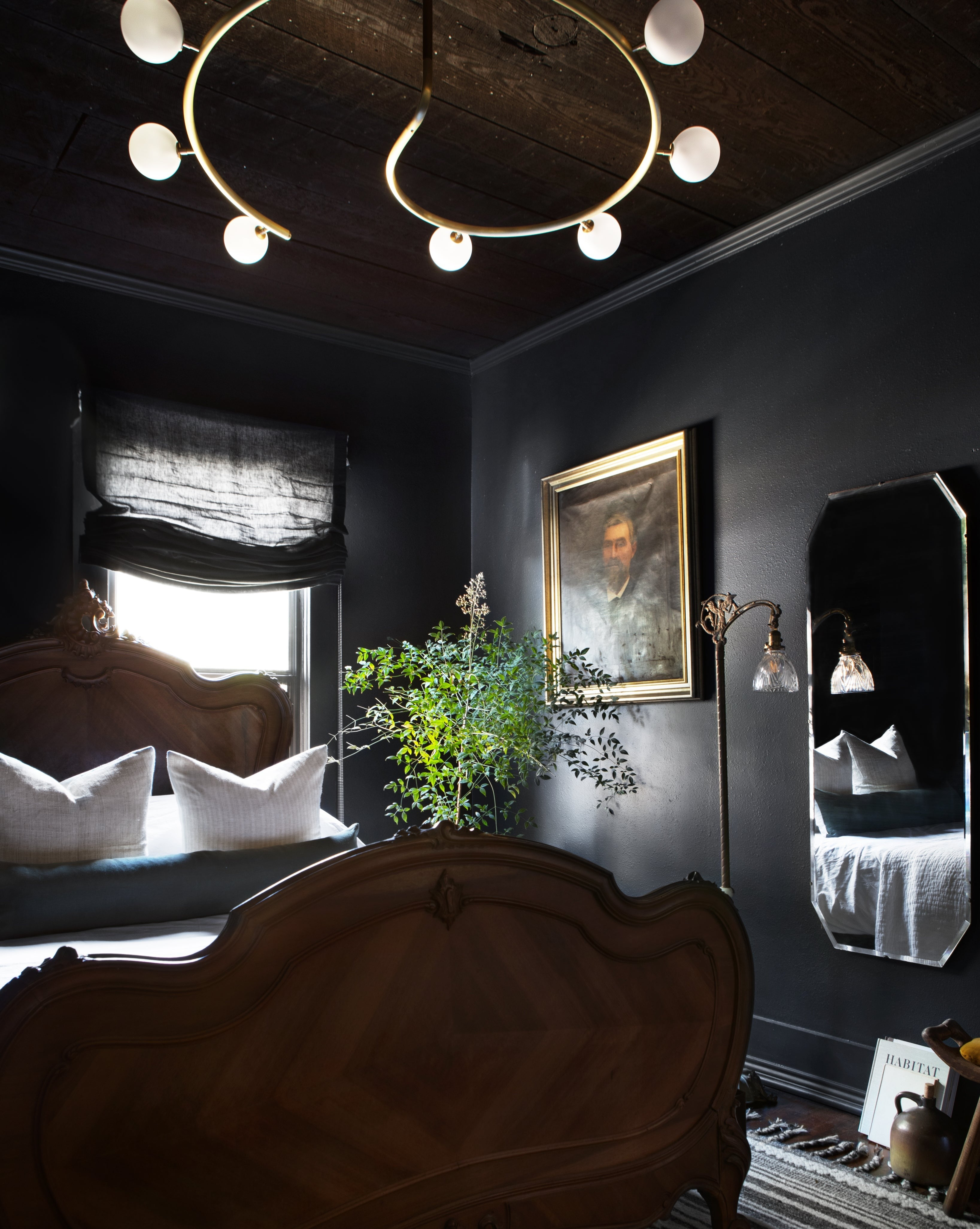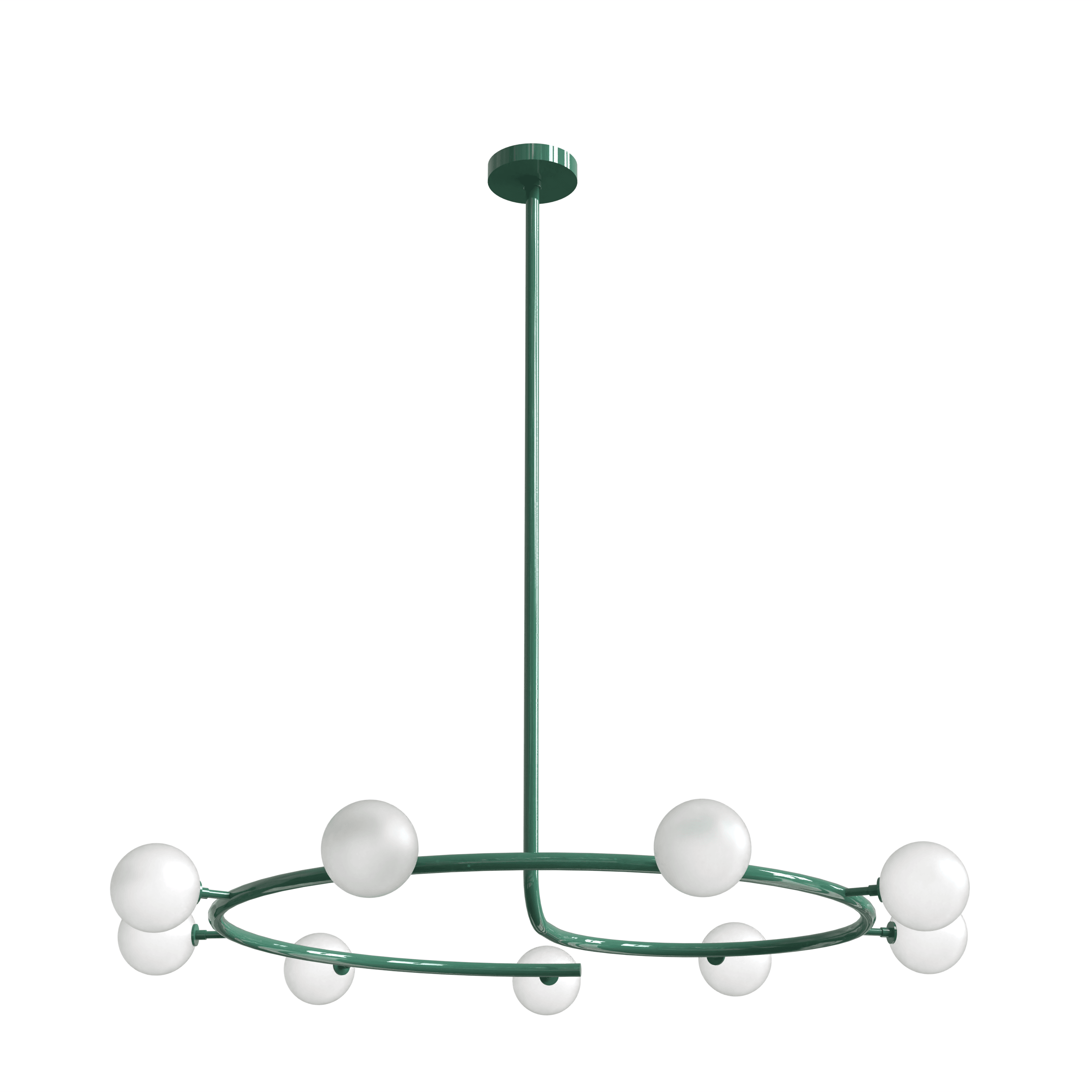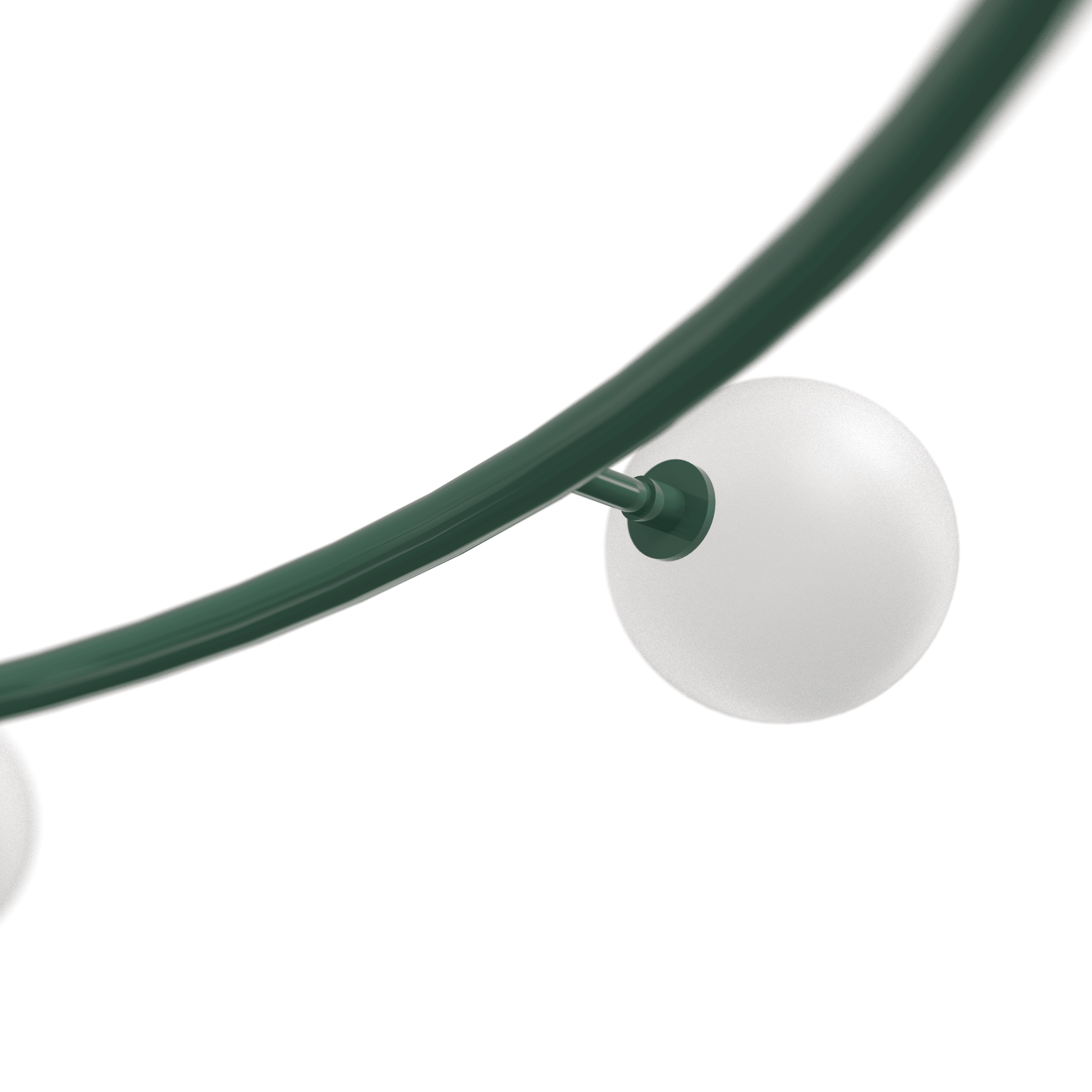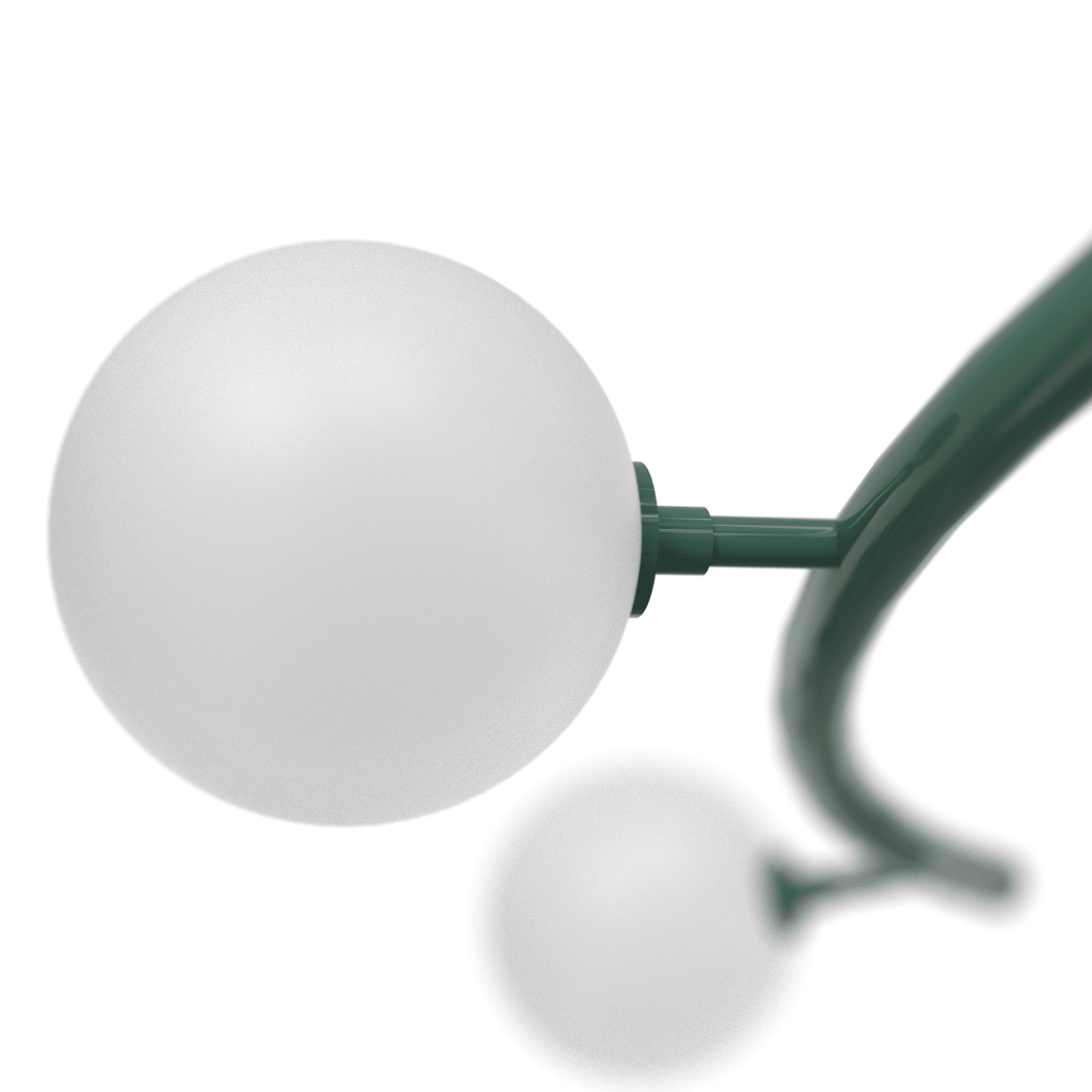Fibonacci (Natural Brass)Fibonacci (Natural Brass) — Interior by Urbanology DesignsFibonacci (Oil Rubbed Bronze) — Interior by Clark + AldineFibonacci (Perfect White)Fibonacci (Oil Rubbed Bronze)Fibonacci (Black)Fibonacci (Paris)Fibonacci (Paris)Fibonacci (Paris)Grand Fibonacci (Pearl), Fibonacci (Paris), Petite Fibonacci (Marigold)

# FIBONACCI

Regular price \$7,695.00
/
The Fibonacci chandelier takes inspiration from the Fibonacci sequence, a mathematical pattern found in nature, art, and architecture. The perfectly balanced spiral pattern of this chandelier captures the beauty and harmony of the Fibonacci spiral, creating a sense of modernity, sophistication, and elegance.

G9 socket (halogen-style socket with 2 pins). Dimmable. We recommend G9 LED bulbs: 5w LED with 60 watt equivalent.

The Fibonacci measures 44" in diameter and includes 9 glass globes fabricated from a matte opaque milk glass. The globes are 4" in diameter and are threaded to screw easily around the socket. Fibonacci is also available in 34" and 54" diameters with 7 and 11 globes, respectively.